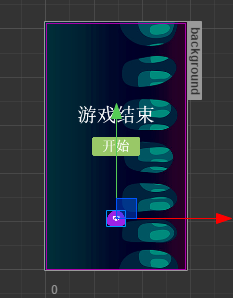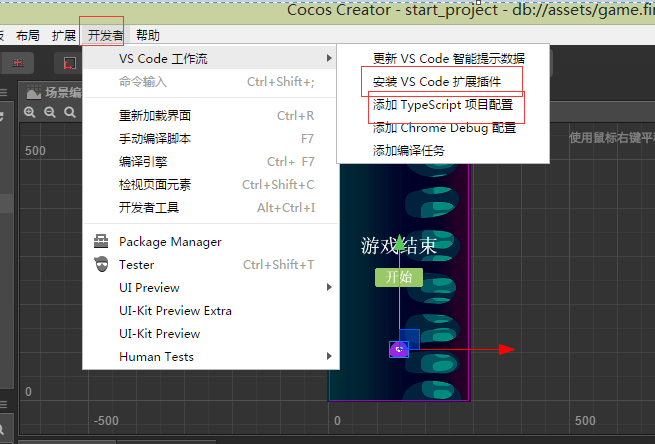# 1. 初来乍到

## 2. 初步探索

cc是一个跨平台框架，一端编译多端发布。想想前端的 mpvue taro uni-app，无不是解决此类问题，再加上gulp,webpack,再来一堆node_modules,啥less sass stylus.各种环境配置那是相当的复杂。所以业界流传，前端已经进入深水区，真的一点不假。 然而cc依然可以让你舒适的写JS或者TS ,并且没有繁杂的配置，一键搞定打包发布。

## 3. 小试牛刀## 4. 一顿操作猛如虎3. update 该回调会频繁调用，每一帧调用一次。对比Vue应该是beforeUpdate回调,虽然他们性质不一样

4. lateUpdate 该回调会频繁调用，也是每帧调用一次，对比Vue应该updated回调

5. onDestroy 根据条件调用，当组件调用了自身的 destroy()方法，会触发此回调

6. onEnable 根据条件调用， enabled 属性从 false 变为 true 或 active 属性从 false 变为 true 触发此回调

7. onDisable 根据条件调用， enabled 属性从 true 变为 false 或active 属性从 true 变为 false触发此回调

## 4.1 让主角动起来

• cc.systemEvent.on(cc.SystemEvent.EventType.KEY_UP, this.onKeyUp, this) 注册一个系统事件 ，支持按键事件和重力感应事件

• this.node.parent.on(cc.Node.EventType.TOUCH_MOVE, this.onTouchMove, this) 在某个节点注册一个Node 支持的事件类型

// author:herbert qq:464884492
onTouchMove(e: cc.Event.EventTouch) {
let deltaX = e.getDeltaX(); //获取本次和上次移动的增量
let deltaY = e.getDeltaY();
//左移
if (deltaX < 0 && this.node.x <= this.leftMinX) return;
if (deltaX > 0 && this.node.x >= this.rightMaxX) return;
if (deltaY > 0 && this.node.y >= this.topMaxY) return;
if (deltaY < 0 && this.node.y <= this.bottomMinY) return;
this.node.x += deltaX;
this.node.y += deltaY;
}

## 4.2 生成坑爹的星星

// author:herbert qq:464884492
buildOneStar() {
let star = cc.instantiate(this.starPrefab);
return star;
}

// author:herbert qq:464884492
buildRandomStar() {
let tempX = 0;
let tempY = 0;
tempX = Math.floor(this.starMaxX - Math.random() * this.starMaxX);//生成一个不大于MaxX的坐标
tempY = Math.floor(this.starMaxY - Math.random() * this.starMaxY);
if (Math.random() < 0.5) tempX = tempX * -1;
let star = this.buildOneStar();
star.setPosition(tempX, tempY);
star.zIndex = this.tank.zIndex - 1;
star.name = "star";
star.getComponent("Star").index = this;
}

start() {
// 定义一个Action
let downAction = cc.moveTo(this.index.starFallSpeed, this.node.x, this.minY - 60);
downAction.easing(cc.easeSineOut());
this.node.runAction(downAction);
}

### 4.3 是时候接住星星了

//author:herbert qq:464884492
...
let distance = this.node.position.sub(this.tank.getPosition()).mag();
if (distance < (this.tank.width / 2 - 5)) {
console.log("接住了");
}
...

## 4.4 来点刺激的

1. 生成星星的速度加快

2. 星星掉落的速度加快

//author:herbert qq:464884492
...
this.index.scoreNum += this.index.starScoreSpeed;
this.index.score.string = "得分:" + this.index.scoreNum;
// 降落速度加
if (Math.floor(this.index.scoreNum / 100) == this.index.starScoreSpeed - 4 && this.index.starFallSpeed > 1) {
this.index.starFallSpeed -= 0.2; //下降速度加快
if (this.index.starBuildTimeOut > 200) {
this.index.starBuildTimeOut -= 100; //生成速度加快
}
this.index.lifeNum += 1;
if (this.index.starScoreSpeed < 10) {
this.index.starScoreSpeed += 1;
}
}
cc.audioEngine.play(this.index.scoreClip, false, 0.2);
this.index.allStars.splice(this.index.allStars.indexOf(this.node), 1)
this.node.destroy();
...

## 4.5 是时候结束了

//author:herbert qq:464884492
...
if (this.node.y <= this.minY) {
this.index.lifeNum -= 1;
this.index.life.string = "生命:" + this.index.lifeNum;
this.node.destroy();
this.index.allStars.splice(this.index.allStars.indexOf(this.node), 1)
if (this.index.lifeNum <= 0) {
this.index.gameOver.node.active = true;
this.index.btnPlay.node.active = true;
this.index.starIsRunning = false;
let storageValue = cc.sys.localStorage.getItem(this.index.HIGHSTORAGEKEY);
if (storageValue && parseInt(storageValue) > this.index.scoreNum) {
return;
}
cc.sys.localStorage.setItem(this.index.HIGHSTORAGEKEY, this.index.scoreNum);
this.index.highScore.string = "最高分:" + this.index.scoreNum;
}
}
...

## 5. 来点实际的

• 开源地址

• 在基本中定义的属性，切记在编辑器中拖动绑定

• 多看官网api，多开实例代码

• 发布微信小游戏一定不要有英文，会导致审核不通过

posted @ 2019-08-06 17:54  _herbert  阅读(...)  评论(...编辑  收藏